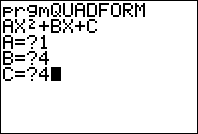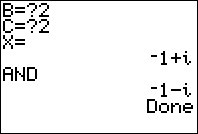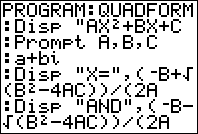# Quadratic Formula Solver for the TI-83+ and TI-84+

by Calcblog
Size: 145 bytes
Downloads: 2055Quadratic Formula Solver for the TI-83+ and TI-84+ 4/5 4 votes

This basic program calculates the roots of a quadratic equation. It is optimized for size to take as little memory as possible. See this blog post for more information on this program

Features:

• Calculates the roots of a second degree polynomial
• Works for polynomials with imaginary roots
• Small file size